# ML Wiki

## Workflow Soundness

Soundness is a notion of correctness of workflow nets

These properties are usually checked with Reachability Graphs

## Situations to Avoid

### Unboundness

Unboundness means:

• there is no bound on the number of tokens that a place can hold
• this always means a problem

### Improper Termination

A workflow net satisfies proper completion when

• if there's a token in the output place, then there are no tokens in other places
• if this property is not satisfied, then the problem is called improper termination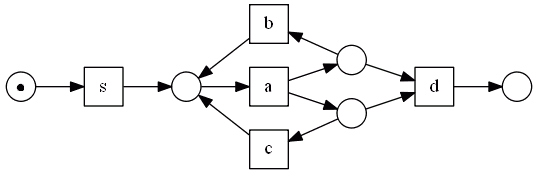• firing sequences:
• $s \to a \to b \to a \to d$
• $s \to a \to c \to a \to d$
• there are remaining tokens after the flow ends
• it means: it stopped, but there is still some work to do
• clearly it's a problem

Example:

•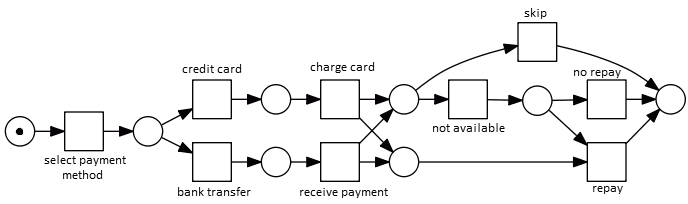• there are paths that lead to proper termination:
• card $\to$ charge $\to$ skip $\to$ finish
• transfer $\to$ receive payment $\to$ NA $\to$ repay
• but there are problematic firing sequences
• card $\to$ charge $\to$ NA $\to$ no repay $\to$ finish
• at the step of no-repay both repay and no repay are available
• after firing no repay there's one token left in the network
•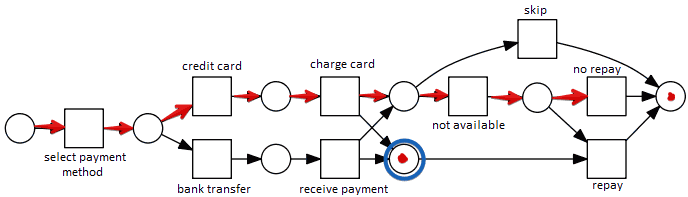Both livelock and deadlock mean that

• there's a situation when you cannot reach the end

•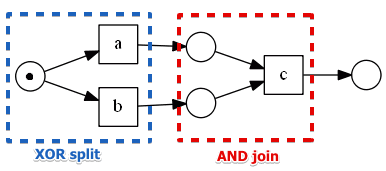• once you fire $a$ nothing is enabled
• but the workflow in not complete: there's no token in the output place
• in this case we don't have matching joins - that leads to a deadlock

Livelock

•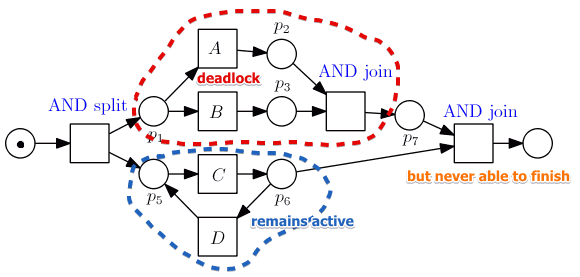• $C$ and $D$ can fire forever: they're always enabled
• but the workflow will never reach the end

• is a transition that can never fire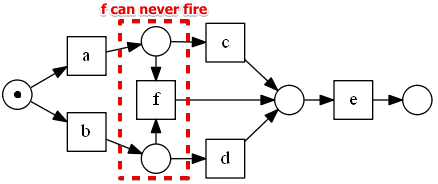• in this case $F$ never gets activated
• this workflow can finish successfully
• the only fix - to remove the dead transition

## Petri Nets

For Petri Nets that represent infinite processes there is a notion of correctness of there nets:

• liveness
• boundness

Used notation:

### Liveness

In a live petri net there are no dead transitions

• a dead transition is a transition that can never fire in any marking reachable from the initial marking

a petri net $N$ with initial marking $M_0$ is live $\iff$

• $\forall M: M_0 \to^* M, \forall t: \exists M \to^* M'$ in which $t$ is enabled

• for any marking $M$ reachable from the initial marking $M_0$
• and for every transition $t \in T$
• there exists a marking $M'$ reachable from $M$ s.t.
• $t$ is enabled in $M'$

In other words:

• no matter what happens, for any $t$ there exists a marking $M'$ reachable from the current state $M$
• such that $t$ is enabled in it

#### Example

•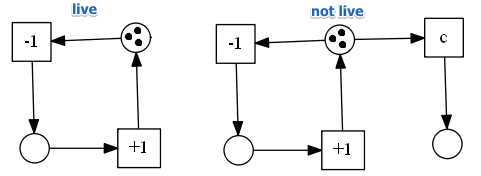• the example on the left is live: no matter what happens the process continues
• the net on the right is not live: after firing $ccc$ nothing is active anymore

#### Example 2

•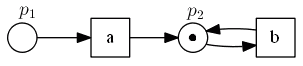• Reachability Graph: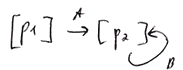• so whatever we do there are always active transitions
• but we cannot escape marking $[p_2]$, and $a$ is no longer live
•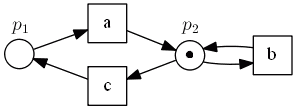• but if we add another transition $c$ it becomes live:
•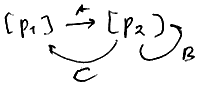So liveness means:

• no matter what happens, every transition $t$ should be able to fire at some point in future

### Boundness

A petri net $N$ with initial marking $M_0$ is $k$-bounded iff

• $\forall M: M_0 \to^* M, \forall p \in P: M(p) \leqslant k$
• there never can appear more than $k$ tokens in any $p \in P$ in any reachable marking $M$

5-bounded net:

• each $p$ can hold no more than 5 tokens

1-bounded net is called safe

#### Example

•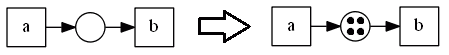• in this net $a$ can fire infinitely many number of times
• so this net is not bounded
• but it's always live: there exists matchings for which $a$ and $b$ can fire

#### Example 2

•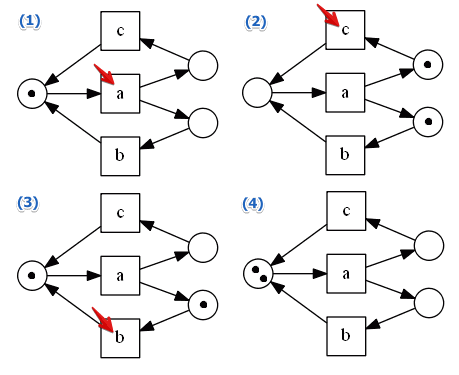• not bounded: can put as many matchings as we want

a petri net $N$ with initial marking $M_0$ is deadlock-free iff

• $\forall M: M_0 \to^* M \ \exists M': M \to M'$
• for any reachable marking $M$ there exists another marking $M'$ that can be reached from $M$
• i.e. every reachable marking enables some transition $t \in T$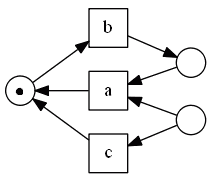• after $b$ fires nothing can fire anymore
• $a$ needs tokens from two its input places, but there's only one token

## Workflow Nets Soundness

With workflow nets

• we can decide many things statically, before enacting the workflow
• there are some desirable properties that help to avoid these problems

A workflow net is sound $\iff$ it has

• the option to complete
• proper completion

Notation:

### Option to Complete

$\forall M, M_0 \to^* M: \exists M', M \to^* M': M' \geqslant [o]$

• for all $M$ reachable from the initial marking
• it should be possible to reach the final marking from $M$

Example:

•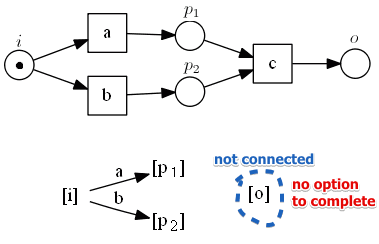• deadlock = no option to complete
• marking $[o]$ is not reachable from $[i]$

Livelock Example:

• consider the net with a livelock
•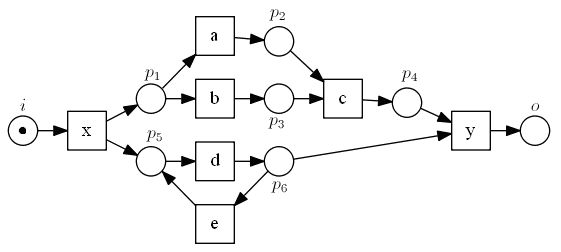• the following is its reachability graph
•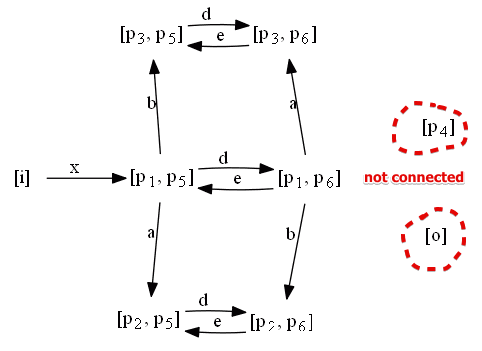• we see that two nodes are not connected: it's clearly a problem

### Proper Completion

$M_0 \to ^* M \land M \geqslant [o] \Rightarrow M \equiv [o]$

• when $M$ is reachable from the initial marking
• and it's great or equal to the final marking
• for proper completion to hold $M$ should be equal to $[o]$

$\forall t \in T, \exists M: M_0 \to^* M$ and $t$ in enabled in $M$

• for all possible transitions there should exists at least one marking $M$ in which $t$ is enabled

### Petri Net Soundness

A sound workflow net can never be live:

• it should be able to reach the final state
• and nothing should be able to fire from that state

A workflow net $N = (P, T, F)$ is sound iff

• a petri net $N' = (P, T \cup \{ \alpha \}, F \cup \{ (o, \alpha), (\alpha, i) \} )$ is sound

So we just connect $o$ and $i$ via some transition $\alpha$

• and check Petri Net soundness
•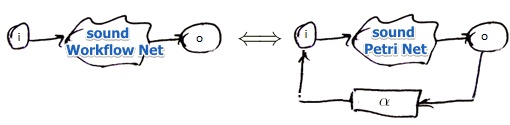Relation:

• if $N$ doesn't have the proper completion property, $N'$ will not be bounded
• if there's a deadlock in $N$,
• there exists a firing sequence in $N'$ s.t.
• it brings you to some state where you cannot reach $o$
• i.e. $N'$ is not live
• dead transitions: same notion in both $N$ and $N'$